• Currently, I am a Machine Learning researcher at Skoltech at Tensor networks and deep learning for applications in data mining laboratory, working with Prof. Ivan Oseledets and Prof. Andrzej Cichocki.

• I received my Ph.D. in Probability Theory and Statistics from Lomonosov Moscow State University, and in parallel, I completed a Master’s-level programm in Computer Science and Data Analysis from the Yandex School of data analysis.

• My recent research deals with compression and acceleration of computer vision models (classification/object detection/segmentation), neural ordinary differential equations, as well as neural networks analysis using low-rank methods, such as tensor decompositions and active subspaces. Also, I have some audio-related activity, particularly, I participate in the project on speech synthesis and voice conversion. Some of my earlier projects were related to medical data processing (EEG, ECG) and included human disease detection, artifact removal, and weariness detection.

• Research interests: deep learning (DL), interpretability of DL, computer vision, speech technologies, multi-modal/multi-task learning, semi-supervised/unsupervised learning, transfer learning, domain adaptation, hyper networks, tensor decompositions for DL.

# Selected publications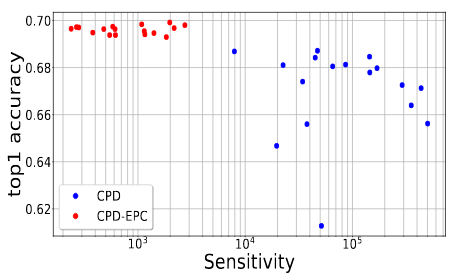Stable Low-rank Tensor Decomposition for Compression of Convolutional Neural Network

A.H. Phan, K. Sobolev, K. Sozykin, D. Ermilov, J. Gusak, P. Tichavsky, V. Glukhov, I. Oseledets, A. Cichocki

ECCV 2020

Most state of the art deep neural networks are overparameterized and exhibit a high computational cost. A straightforward approach to this problem is to replace convolutional kernels with its low-rank tensor approximations, whereas the Canonical Polyadic tensor Decomposition is one of the most suited models. However, fitting the convolutional tensors by numerical optimization algorithms often encounters diverging components, i.e., extremely large rank-one tensors but canceling each other. Such degeneracy often causes the non-interpretable result and numerical instability for the neural network fine-tuning. This paper is the first study on degeneracy in the tensor decomposition of convolutional kernels. We present a novel method, which can stabilize the low-rank approximation of convolutional kernels and ensure efficient compression while preserving the high-quality performance of the neural networks. We evaluate our approach on popular CNN architectures for image classification and show that our method results in much lower accuracy degradation and provides consistent performance.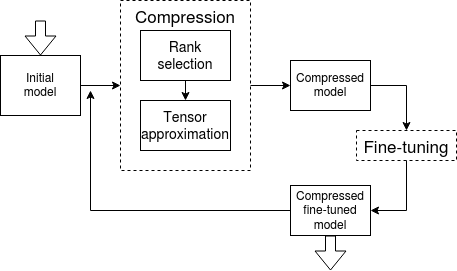Automated Multi-Stage Compression of Neural Networks

J. Gusak, M. Kholiavchenko, E. Ponomarev, L. Markeeva, A. Cichocki, I. Oseledets

ICCV 2019 Workshop on Low-Power Computer Vision

We propose a new simple and efficient iterative approach for compression of deep neural networks, which alternates low-rank factorization with smart rank selection and fine-tuning. We demonstrate the efficiency of our method comparing to non-iterative ones. Our approach improves the compression rate while maintaining the accuracy for a variety of computer vision tasks.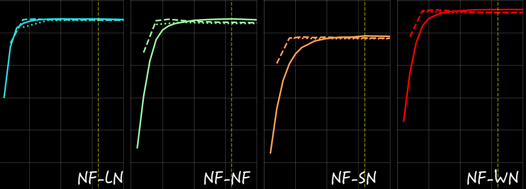Towards Understanding Normalization in Neural ODEs

J. Gusak, L. Markeeva, T. Daulbaev, A. Katrutsa, A. Cichocki, I. Oseledets

ICLR 2020 DeepDiffeq workshop

Normalization is an important and vastly investigated technique in deep learning. However, its role for Ordinary Differential Equation based networks (neural ODEs) is still poorly understood. This paper investigates how different normalization techniques affect the performance of neural ODEs. Particularly, we show that it is possible to achieve 93% accuracy in the CIFAR-10 classification task, and to the best of our knowledge, this is the highest reported accuracy among neural ODEs tested on this problem.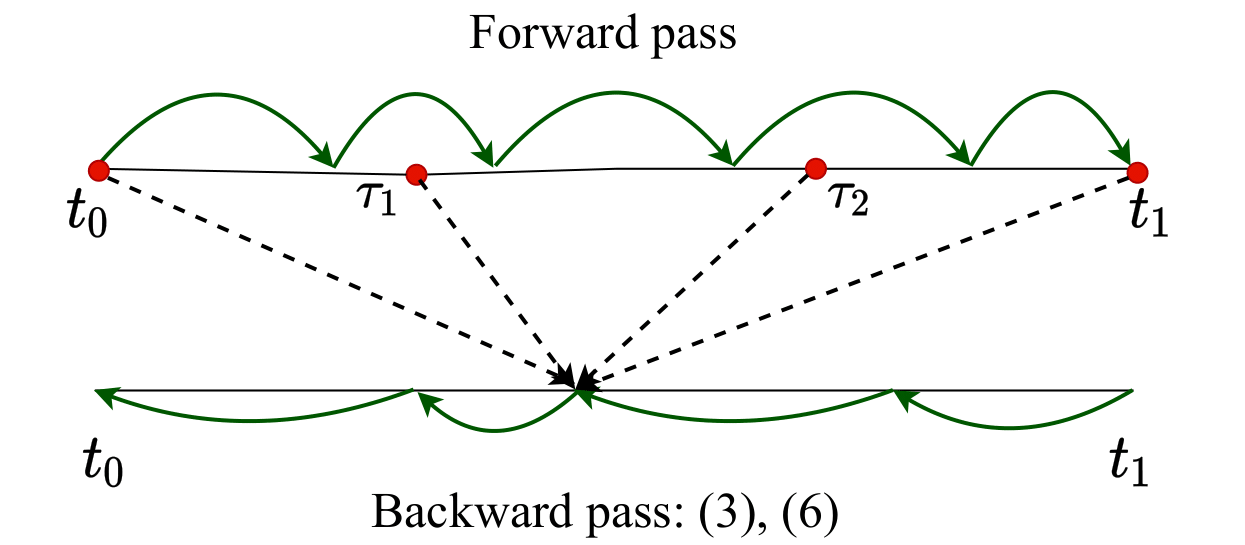Interpolation technique to speed up gradients propagation in neural ordinary differential equations

T. Daulbaev, A. Katrutsa, L. Markeeva, J. Gusak, A. Cichocki, I. Oseledets

NeurIPS 2020

We propose a simple interpolation-based method for the efficient approximation of gradients in neural ODE models. We compare it with reverse dynamic method (known in literature as ''adjoint method'') to train neural ODEs on classification, density estimation and inference approximation tasks. We also propose a theoretical justification of our approach using logarithmic norm formalism. As a result, our method allows faster model training than reverse dynamic method on several standard benchmarks.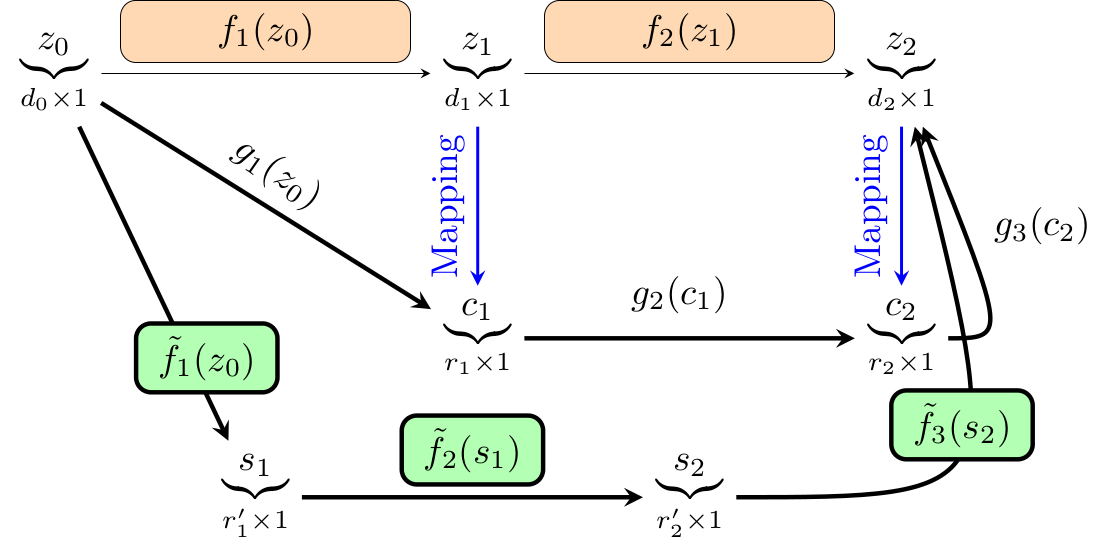Reduced-Order Modeling of Deep Neural Networks

J. Gusak, T. Daulbaev E. Ponomarev, A. Cichocki, I. Oseledets

arxiv 2019 (accepted to Computational Mathematics and Mathematical Physics Journal)

We introduce a new method for speeding up the inference of deep neural networks. It is somewhat inspired by the reduced-order modeling techniques for dynamical systems. The cornerstone of the proposed method is the maximum volume algorithm. We demonstrate efficiency on VGG and ResNet architectures pre-trained on different datasets. We show that in many practical cases it is possible to replace convolutional layers with much smaller fully-connected layers with a relatively small drop in accuracy.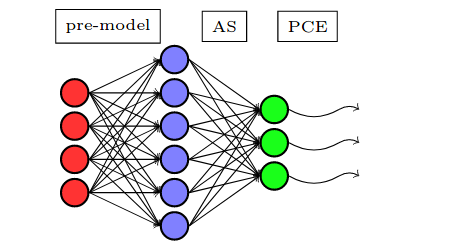Active Subspace of Neural Networks: Structural Analysis and Universal Attacks

C. Cui, K. Zhang, T. Daulbaev, J. Gusak, I. Oseledets, Z. Zhang

arxiv 2019 (accepted to SIAM Journal on Mathematics of Data Science, SIMODS)

Active subspace is a model reduction method widely used in the uncertainty quantification community. Firstly, we employ the active subspace to measure the number of" active neurons" at each intermediate layer and reduce the number of neurons from several thousands to several dozens, yielding to a new compact network. Secondly, we propose analyzing the vulnerability of a neural network using active subspace and finding an additive universal adversarial attack vector that can misclassify a dataset with a high probability.

# Selected projectsData loaders for speech and audio data sets

A Python library with PyTorch and TFRecords data loaders for convenient preprocessing of popular speech, music and environmental sound data sets.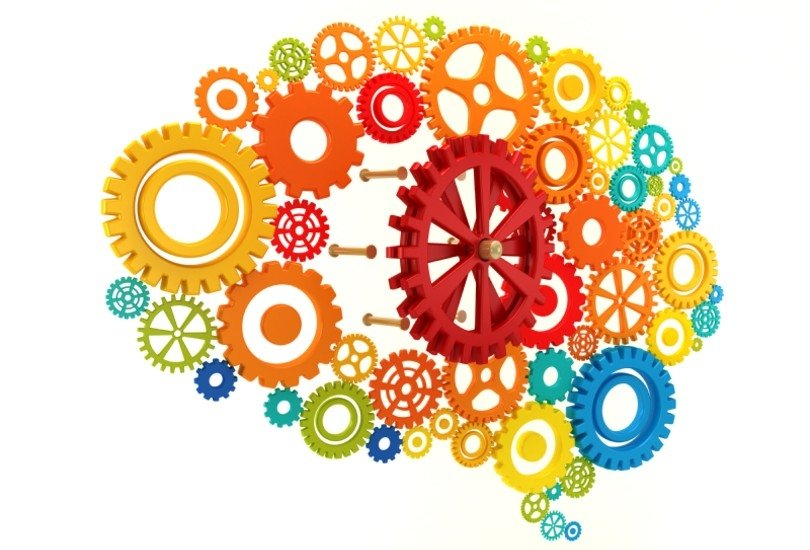FlopCo: FLOP and other statistics COunter for Pytorch neural networks

A Python library FlopCo has been created to make FLOP and MAC counting simple and accessible for Pytorch neural networks. Moreover, FlopCo allows to collect other useful model statistics, such as number of parameters, shapes of layer inputs/outputs, etc.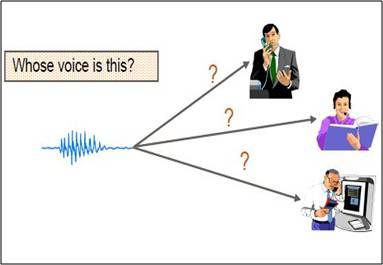Speaker identification using neural network models

In the framework of this project a WaveNet-style autoencoder model for audio synthesis and a neural network for environment sounds classification have been adopted to solve speaker identification task.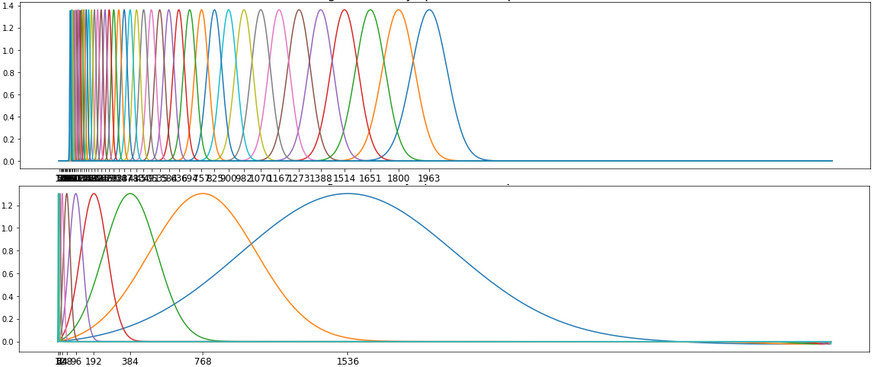Scattering transform

Python implementation of the scattering transform from MATLAB toolbox ScatNet.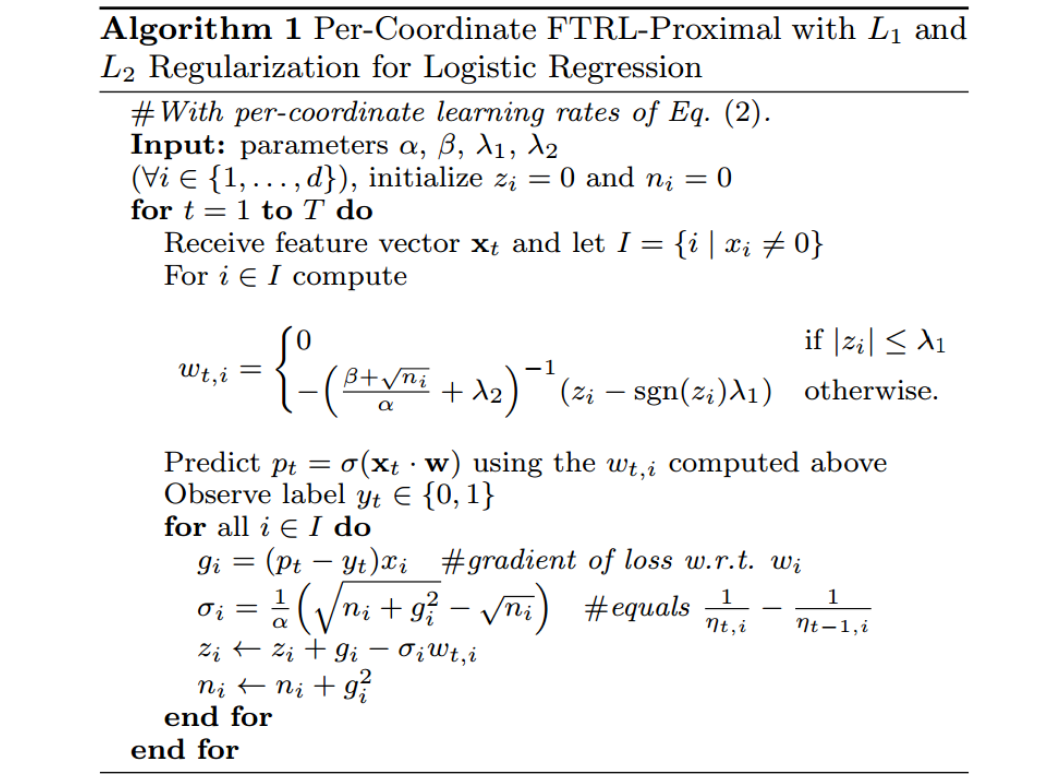Per-Coordinate FTRL-Proximal with L1 and L2 Regularization for Logistic Regression

C++ implementation of the online optimization algorithm for logistic regression training, described in the following paper.